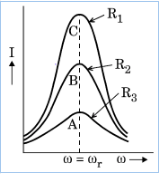# (a) What do you understand by 'sharpness of resonance'for a series LCR resonant circuit? How is it related with the quality factor 'Q'of the circuit? using the graphs given in the diagram, explain the factors which affect it. For which graph is the resistance (R) minimum?(b) A2 F capacitor,100  resistor and 8 H inductor are connected in series with an ac source. Find the frequency of the ac source for which the current drawn in the circuit is maximum.If the peak value of emf of the source is 200 V, calculate the (i)maximum current,and(ii) inductive and capacitive reactance of the circuit at resonance.

(a) The circuit would be set to have a high sharpness of Resonance if the current in the circuit drops rapidly as the frequency of the applied AC Source shifts from its resonant value.
The sharpness of Resonance is measured by the quality factor(Q).

R is minimum for C
(b) we have given;
The frequency

where, L is inductor and
C is Capacitor

(i)calculating the maximum current;
we have

At resonance Z=R

(ii)To determine the inductive and Capacitance reactance;
we know:
at resonance

on putting the values we have,

### Preparation Products

##### Knockout NEET Sept 2020

An exhaustive E-learning program for the complete preparation of NEET..

₹ 15999/- ₹ 6999/-
##### Rank Booster NEET 2020

This course will help student to be better prepared and study in the right direction for NEET..

₹ 9999/- ₹ 4999/-
##### Knockout JEE Main Sept 2020

An exhaustive E-learning program for the complete preparation of JEE Main..

₹ 12999/- ₹ 6999/-
##### Test Series NEET Sept 2020

Take chapter-wise, subject-wise and Complete syllabus mock tests and get in depth analysis of your test..

₹ 4999/- ₹ 2999/-